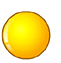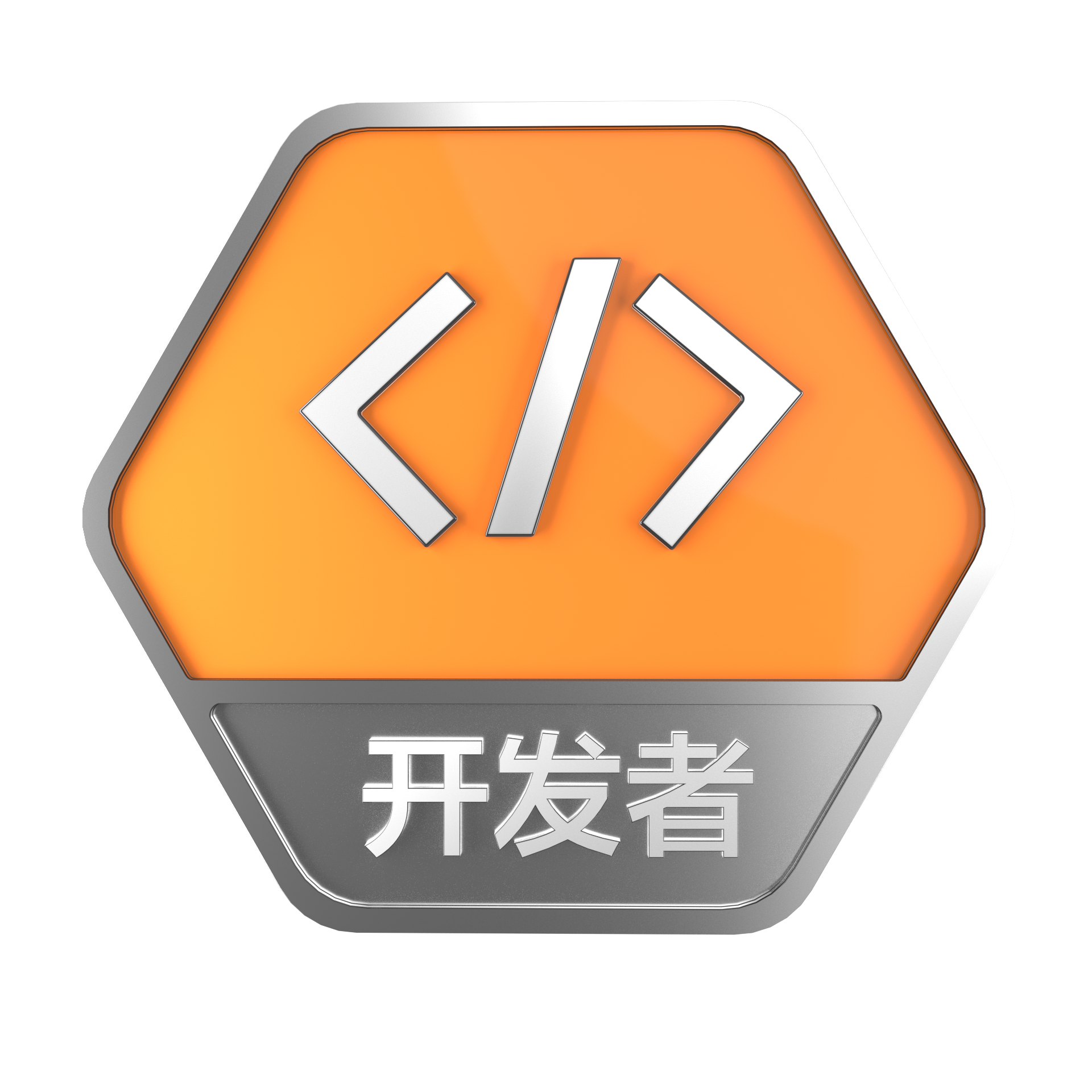# PHP引用(&)使用详解

+关注继续查看

php的引用（就是在变量或者函数、对象等前面加上&符号）

1.变量的引用

PHP 的引用允许你用两个变量来指向同一个内容

<?

$a="ABC";$b =&$a; echo$a;//这里输出:ABC
echo $b;//这里输出:ABC$b="EFG";

echo $a;//这里$a的值变为EFG 所以输出EFG
echo $b;//这里输出EFG ?> 2.函数的引用传递（传址调用 传址调用我就不多说了 下面直接给出代码 <?php function test(&$a)
{

$a=$a+100;
}

$b=1; echo$b;//输出１
test($b); //这里$b传递给函数的其实是$b的变量内容所处的内存地址，通过在函数里改变$a的值　就可以改变$b的值了 echo "<br>"; echo$b;//输出101
?>

上面的“ test($b); ” 中的$b前面不要加 & 符号，但是在函数“call_user_func_array”中，若要引用传参，就得需要 & 符号，如下代码所示：

3.函数的引用返回

<?php
function &test()
{

static $b=0;//申明一个静态变量$b=$b+1; echo$b;

return $b; }$a=test();//这条语句会输出　$b的值 为１$a=5;
$a=test();//这条语句会输出$b的值　为2

$a=&test();//这条语句会输出$b的值　为3
$a=5;$a=test();//这条语句会输出　$b的值 为6 ?> 下面解释下： 通过这种方式$a=test();得到的其实不是函数的引用返回，这跟普通的函数调用没有区别　至于原因：　这是ＰＨＰ的规定
ＰＨＰ规定通过$a=&test(); 方式得到的才是函数的引用返回 至于什么是引用返回呢（ＰＨＰ手册上说：引用返回用在当想用函数找到引用应该被绑定在哪一个变量上面时。) 这句狗屁话 害我半天没看懂 用上面的例子来解释就是$a=test()方式调用函数，只是将函数的值赋给$a而已， 而$a做任何改变　都不会影响到函数中的$b 而通过$a=&test()方式调用函数呢, 他的作用是　将return $b中的$b变量的内存地址与$a变量的内存地址 指向了同一个地方 即产生了相当于这样的效果($a=&$b;) 所以改变$a的值　也同时改变了$b的值 所以在执行了$a=&test();
$a=5; 以后，$b的值变为了5

This is the way how we use pointer to access variable inside the class.

<?php
class talker{

private $data = 'Hi'; public function & get(){ return$this->data;
}

public function out(){

echo $this->data; } }$aa = new talker();
$d = &$aa->get();

$aa->out();$d = 'How';
$aa->out();$d = 'Are';
$aa->out();$d = 'You';
$aa->out(); ?> the output is "HiHowAreYou" 4.对象的引用 <?php class a{ var$abc="ABC";
}

$b=new a;$c=$b; echo$b->abc;//这里输出ABC
echo $c->abc;//这里输出ABC$b->abc="DEF";

echo $c->abc;//这里输出DEF ?> 以上代码是在PHP5中的运行效果 在PHP5中 对象的赋值是个引用的过程。上列中$b=new a; $c=$b; 其实等效于$b=new a;$c=&$b; PHP5中默认就是通过引用来调用对象， 但有时你可能想建立一个对象的副本，并希望原来的对象的改变不影响到副本 . 为了这样的目的，PHP5定义了一个特殊的方法，称为__clone 自 PHP 5 起，new 自动返回引用，因此在此使用 =& 已经过时了并且会产生 E_STRICT 级别的消息。 在php4中，对象的赋值是个拷贝过程， 如：$b=new a，其中new a产生的是一个匿名的a对象实例，而此时的$b是对这个匿名对象的拷贝。同理$c=$b，也是对$b内容的一个拷贝。所以在php4中，为了节省内存空间，$b=new a 一般会改成引用的模式，即$b=& new a。

在php5中，你不需要额外添加什么东西就可到达“对象引用”的功能：

<?php
class foo{

protected $name; function __construct($str){

$this->name =$str;
}

function __toString(){

return  'my name is "'. $this->name .'" and I live in "' . __CLASS__ . '".' . "\n"; } function setName($str){

$this->name =$str;
}
}

class MasterOne{

protected $foo; function __construct($f){

$this->foo =$f;
}

function __toString(){

return 'Master: ' . __CLASS__ . ' | foo: ' . $this->foo . "\n"; } function setFooName($str){

$this->foo->setName($str );
}
}

class MasterTwo{

protected $foo; function __construct($f){

$this->foo =$f;
}

function __toString(){

return 'Master: ' . __CLASS__ . ' | foo: ' . $this->foo . "\n"; } function setFooName($str){

$this->foo->setName($str );
}
}

$bar = new foo('bar'); print("\n"); print("Only Created \$bar and printing \$bar\n"); print$bar );

print("\n");
print("Now \$baz is referenced to \$bar and printing \$bar and \$baz\n");
$baz =&$bar;
print$bar ); print("\n"); print("Now Creating MasterOne and Two and passing \$bar to both constructors\n");
$m1 = new MasterOne($bar );
$m2 = new MasterTwo($bar );
print$m1 ); print$m2 );

print("\n");
print("Now changing value of \$bar and printing \$bar and \$baz\n");$bar->setName('baz');
print$bar ); print$baz );

print("\n");
print("Now printing again MasterOne and Two\n");
print$m1 ); print$m2 );

print("\n");
print("Now changing MasterTwo's foo name and printing again MasterOne and Two\n");
$m2->setFooName( 'MasterTwo\'s Foo' ); print$m1 );
print$m2 ); print("Also printing \$bar and \$baz\n"); print$bar );
print$baz ); ?> 输出： Only Created$bar and printing $bar my name is "bar" and I live in "foo". Now$baz is referenced to $bar and printing$bar and $baz my name is "bar" and I live in "foo". Now Creating MasterOne and Two and passing$bar to both constructors
Master
: MasterOne | foo: my name is "bar" and I live in "foo".

Master
: MasterTwo | foo: my name is "bar" and I live in "foo".

Now changing value of
$bar and printing$bar and $baz my name is "baz" and I live in "foo". my name is "baz" and I live in "foo". Now printing again MasterOne and Two Master : MasterOne | foo: my name is "baz" and I live in "foo". Master : MasterTwo | foo: my name is "baz" and I live in "foo". Now changing MasterTwo 's foo name and printing again MasterOne and Two Master: MasterOne | foo: my name is "MasterTwo 's Foo" and I live in "foo". Master: MasterTwo | foo: my name is "MasterTwo's Foo" and I live in "foo". Also printing$bar and $baz my name is "MasterTwo 's Foo" and I live in "foo". my name is "MasterTwo's Foo" and I live in "foo". 上个例子解析：$bar = new foo('bar');
$m1 = new MasterOne($bar );
$m2 = new MasterTwo($bar );

1.$m1或$m2内部，任何对$bar的操作都会影响外部对象实例$bar的相关值。
2.外部对象实例$bar的改变也会影响$m1和$m2内部的$bar的引用相关值。

class foo{

var $bar; function setBar(&$newBar){

$this->bar =& newBar; } } 5.引用的作用 如果程序比较大,引用同一个对象的变量比较多,并且希望用完该对象后手工清除它,个人建议用 "&" 方式,然后用$var=null的方式清除. 其它时候还是用php5的默认方式吧. 另外, php5中对于大数组的传递,建议用 "&" 方式, 毕竟节省内存空间使用。

6.取消引用

<?php

$a = 1;$b =& $a; unset ($a);
?>

7.global 引用

$var2 = ""; function global_references($use_globals)
{

global $var1,$var2;

if (!$use_globals) {$var2 =& $var1// visible only inside the function } else {$GLOBALS["var2"=& $var1// visible also in global context } } global_references( false); echo "var2 is set to '$var2'\n"// var2 is set to ''
global_references(true);
echo "var2 is set to '$var2'\n"// var2 is set to 'Example variable' ?> global$var; 当成是 $var =&$GLOBALS['var']; 的简写。从而将其它引用赋给 $var 只改变了本地变量的引用。 8.$this

$b=&$a;
?>

２:如果在上面的代码基础上再加上如下代码

<?php

$a="EFG"; ?> 由于$a与$b所指向的内存的数据要重新写一次了，此时Zend核心会自动判断 自动为$b生产一个$a的数据拷贝，重新申请一块内存进行存储 php的引用（就是在变量或者函数、对象等前面加上&符号）是个高级话题，新手多注意，正确的理解php的引用很重要，对性能有较大影响，而且理解错误可能导致程序错误！ 很 多人误解php中的引用跟C当中的指针一样，事实上并非如此，而且很大差别。C语言中的指针除了在数组传递过程中不用显式申明外，其他都需要使用*进行定 义，而php中对于地址的指向（类似指针）功能不是由用户自己来实现的，是由Zend核心实现的，php中引用采用的是“写时拷贝”的原理，就是除非发生 写操作，指向同一个地址的变量或者对象是不会被拷贝的，比如下面的代码：$a = array('a','c'...'n');
$b =$a;

function printArray(&$arr//引用传递 { print(count($arr));

}
printArray(
$a); 上面的代码中，我们通过引用把$a数组传入printArray()函数，zend引擎会认为printArray()可能会导致对$a的改变，此时就会自动为$b生产一个$a的数据拷贝，重新申请一块内存进行存储。这就是前面提到的“写时拷贝”概念。 如果我们把上面的代码改成下面这样： function printArray($arr)   //值传递
{

print(count($arr)); } printArray($a);

<?php $bar =& new fooclass();$foo =& find_var($bar);?>  自 PHP 5 起，new 自动返回引用，因此在此使用 =& 已经过时了并且会产生 E_STRICT 级别的消息。 Note: 不用 & 运算符导致对象生成了一个拷贝。如果在类中用$this，它将作用于该类当前的实例。没有用 & 的赋值将拷贝这个实例（例如对象）并且 $this 将作用于这个拷贝上，这并不总是想要的结果。由于性能和内存消耗的问题，通常只想工作在一个实例上面。 尽管可以用 @ 运算符来抑制构造函数中的任何错误信息，例如用 @new，但用 &new 语句时这不起效果。这是 Zend 引擎的一个限制并且会导致一个解析错误。 Warning 如果在一个函数内部给一个声明为 global 的变量赋于一个引用，该引用只在函数内部可见。可以通过使用$GLOBALS 数组避免这一点。

Example #2 在函数内引用全局变量

<?php $var1 = "Example variable";$var2 = ""; function global_references($use_globals) { global$var1, $var2; if (!$use_globals) {         $var2 =&$var1; // visible only inside the function     } else {         $GLOBALS["var2"] =&$var1; // visible also in global context     } }global_references(false); echo "var2 is set to '$var2'\n"; // var2 is set to ''global_references(true); echo "var2 is set to '$var2'\n"; // var2 is set to 'Example variable'?> 
global $var; 当成是$var =& $GLOBALS['var']; 的简写。从而将其它引用赋给$var 只改变了本地变量的引用。

Note:

Example #3 引用与 foreach 语句

<?php $ref = 0;$row =& $ref; foreach (array(1, 2, 3) as$row) {     // do something} echo $ref; // 3 - last element of the iterated array?>  引用做的第二件事是用引用传递变量。这是通过在函数内建立一个本地变量并且该变量在呼叫范围内引用了同一个内容来实现的。例如： <?phpfunction foo(&$var) {     $var++; }$a=5;foo($a);?>  将使$a 变成 6。这是因为在 foo 函数中变量 $var 指向了和$a 指向的同一个内容。更多详细解释见引用传递数组引用的一个bug（后来仔细推敲，其实不是bug）

It appears that references can have side-effects.  Below are two examples.  Both are simply copying one array to another.  In the second example, a reference is made to a value in the first array before the copy.  In the first example the value at index 0 points to two separate memory locations. In the second example, the value at index 0 points to the same memory location. I won't say this is a bug, because I don't know what the designed behavior of PHP is, but I don't think ANY developers would expect this behavior, so look out. An example of where this could cause problems is if you do an array copy in a script and expect on type of behavior, but then later add a reference to a value in the array earlier in the script, and then find that the array copy behavior has unexpectedly changed.
<?php
// Example one
$arr1 = array(1); echo "\nbefore:\n"; echo "\$arr1 == {$arr1}\n";$arr2 = $arr1;$arr2++;
echo "\nafter:\n";
echo "\$arr1 == {$arr1}\n";
echo "\$arr2 == {$arr2}\n";

before:
$arr1 == 1 after:$arr1 == 1
$arr2 == 2 // Example two$arr3=array(1);
$a=&$arr3;
echo"\nbefore:\n";
echo"\$a ==$a\n";
echo"\$arr3 == {$arr3}\n";
$arr4=$arr3;
$arr4++; echo"\nafter:\n"; echo"\$a == $a\n"; echo"\$arr3 == {$arr3}\n"; echo"\$arr4 == {$arr4}\n"; 输出： before:$a == 1
$arr3 == 1 after:$a == 2
$arr3 == 2$arr4 == 2

?>

对于“Example two”，刚开始还以为是个bug，其实仔细推敲，非也，分析如下，

$arr4=$arr3;1. 什么是引用 在 PHP 中引用是指用不同的名字访问同一个变量内容。 PHP 中的变量名和变量内容是不一样的， 因此同样的内容可以有不同的名字。 最接近的比喻是 Unix 的文件名和文件本身——变量名是目录条目，而变量内容则是文件本身。引用可以被看作是 Unix 文件系统中的硬链接。 PHP 中的引用并不像 C 的指针：例如你不能对他们做指针运算。引用并不是实际的内存地址，而是符号表别名。
31 0php mysql 异步, php mysql 异步并发查询
php mysql 异步, php mysql 异步并发查询
54 0PHP连接MySQL 8.0报错的解决办法
PHP连接MySQL 8.0报错的解决办法
195 0《PHP和MySQL Web开发从新手到高手（第5版）》一一2.1 数据库简介
1705 0《PHP和MySQL Web开发从新手到高手（第5版）》一一第2章　MySQL简介
1450 0《PHP和MySQL Web开发从新手到高手（第5版）》一一1.7 万事俱备，摩拳擦掌
1568 0《PHP和MySQL Web开发从新手到高手（第5版）》一一1.6 第一个PHP脚本
1936 0centos下 Apache、php、mysql默认安装路径
apache: 如果采用RPM包安装，安装路径应在 /etc/httpd目录下 apache配置文件:/etc/httpd/conf/httpd.conf Apache模块路径：/usr/sbin/apachectl web目录:/var/www/html 如果采用源代码安装，一般默认安装在/usr/local/apache2目录下 php: 如果采用RPM包安装，安装路
3029 01343 0windows2003目录权限设置(iis+php+mysql)
http://hi.baidu.com/aquany/blog/item/81e199a238b6faa9cbefd0f9.
618 0+关注
374

5